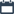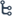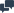## Wednesday 26 April 2023

•April 26, 2023
•Unknown
•, , , ,
•No comments

# Simplification Important Questions

Simplification problems are mathematical problems that involve simplifying an expression by performing arithmetic operations such as addition, subtraction, multiplication, and division, as well as other mathematical operations such as exponents, roots, and percentages. The aim of simplification problems is to arrive at a single, simplified expression that represents the same value as the original expression.

Simplification problems are commonly found in various competitive exams, including the TNPSC Maths exam. They require a good understanding of basic arithmetic concepts and mathematical rules, as well as the ability to solve problems quickly and accurately.

To solve simplification problems, it is essential to follow the correct order of operations, also known as the BODMAS rule. This rule states that we should perform operations in the following order: parentheses, exponents, multiplication and division (from left to right), and addition and subtraction (from left to right).